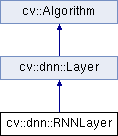OpenCV  3.4.0 Open Source Computer Vision
cv::dnn::RNNLayer Class Referenceabstract

Classical recurrent layer. More...

#include "all_layers.hpp"

Inheritance diagram for cv::dnn::RNNLayer:## Public Member Functions

virtual void setProduceHiddenOutput (bool produce=false)=0
If this flag is set to true then layer will produce $$h_t$$ as second output. More...

virtual void setWeights (const Mat &Wxh, const Mat &bh, const Mat &Whh, const Mat &Who, const Mat &bo)=0Public Member Functions inherited from cv::dnn::Layer
Layer ()

Layer (const LayerParams &params)
Initializes only name, type and blobs fields. More...

virtual ~Layer ()

virtual void applyHalideScheduler (Ptr< BackendNode > &node, const std::vector< Mat *> &inputs, const std::vector< Mat > &outputs, int targetId) const
Automatic Halide scheduling based on layer hyper-parameters. More...

virtual void finalize (const std::vector< Mat *> &input, std::vector< Mat > &output)
Computes and sets internal parameters according to inputs, outputs and blobs. More...

void finalize (const std::vector< Mat > &inputs, std::vector< Mat > &outputs)

std::vector< Matfinalize (const std::vector< Mat > &inputs)

virtual void forward (std::vector< Mat *> &input, std::vector< Mat > &output, std::vector< Mat > &internals)=0
Given the input blobs, computes the output blobs. More...

virtual void forward (InputArrayOfArrays inputs, OutputArrayOfArrays outputs, OutputArrayOfArrays internals)=0
Given the input blobs, computes the output blobs. More...

void forward_fallback (InputArrayOfArrays inputs, OutputArrayOfArrays outputs, OutputArrayOfArrays internals)
Given the input blobs, computes the output blobs. More...

virtual int64 getFLOPS (const std::vector< MatShape > &inputs, const std::vector< MatShape > &outputs) const

virtual bool getMemoryShapes (const std::vector< MatShape > &inputs, const int requiredOutputs, std::vector< MatShape > &outputs, std::vector< MatShape > &internals) const

virtual Ptr< BackendNodeinitHalide (const std::vector< Ptr< BackendWrapper > > &inputs)
Returns Halide backend node. More...

virtual int inputNameToIndex (String inputName)
Returns index of input blob into the input array. More...

virtual int outputNameToIndex (String outputName)
Returns index of output blob in output array. More...

void run (const std::vector< Mat > &inputs, std::vector< Mat > &outputs, std::vector< Mat > &internals)
Allocates layer and computes output. More...

virtual bool setActivation (const Ptr< ActivationLayer > &layer)
Tries to attach to the layer the subsequent activation layer, i.e. do the layer fusion in a partial case. More...

virtual bool setBatchNorm (const Ptr< BatchNormLayer > &layer)
Tries to attach to the layer the subsequent batch normalization layer, i.e. do the layer fusion in a partial case. More...

void setParamsFrom (const LayerParams &params)
Initializes only name, type and blobs fields. More...

virtual bool setScale (const Ptr< ScaleLayer > &layer)
Tries to attach to the layer the subsequent scaling layer, i.e. do the layer fusion in a partial case. More...

virtual bool supportBackend (int backendId)
Ask layer if it support specific backend for doing computations. More...

virtual Ptr< BackendNodetryAttach (const Ptr< BackendNode > &node)
Implement layers fusing. More...

virtual void unsetAttached ()
"Deattaches" all the layers, attached to particular layer. More...Public Member Functions inherited from cv::Algorithm
Algorithm ()

virtual ~Algorithm ()

virtual void clear ()
Clears the algorithm state. More...

virtual bool empty () const
Returns true if the Algorithm is empty (e.g. in the very beginning or after unsuccessful read. More...

virtual String getDefaultName () const

virtual void read (const FileNode &fn)
Reads algorithm parameters from a file storage. More...

virtual void save (const String &filename) const

virtual void write (FileStorage &fs) const
Stores algorithm parameters in a file storage. More...

void write (const Ptr< FileStorage > &fs, const String &name=String()) const
simplified API for language bindings This is an overloaded member function, provided for convenience. It differs from the above function only in what argument(s) it accepts. More...

## Static Public Member Functions

static Ptr< RNNLayercreate (const LayerParams &params)Static Public Member Functions inherited from cv::Algorithm
template<typename _Tp >
static Ptr< _Tp > load (const String &filename, const String &objname=String())
Loads algorithm from the file. More...

template<typename _Tp >
static Ptr< _Tp > loadFromString (const String &strModel, const String &objname=String())
Loads algorithm from a String. More...

template<typename _Tp >
static Ptr< _Tp > read (const FileNode &fn)
Reads algorithm from the file node. More...Public Attributes inherited from cv::dnn::Layer
std::vector< Matblobs
List of learned parameters must be stored here to allow read them by using Net::getParam(). More...

String name
Name of the layer instance, can be used for logging or other internal purposes. More...

int preferableTarget
prefer target for layer forwarding More...

String type
Type name which was used for creating layer by layer factory. More...Protected Member Functions inherited from cv::Algorithm
void writeFormat (FileStorage &fs) const

## Detailed Description

Classical recurrent layer.

Accepts two inputs $$x_t$$ and $$h_{t-1}$$ and compute two outputs $$o_t$$ and $$h_t$$.

• input: should contain packed input $$x_t$$.
• output: should contain output $$o_t$$ (and $$h_t$$ if setProduceHiddenOutput() is set to true).

input should have shape [T, N, data_dims] where T and N is number of timestamps and number of independent samples of $$x_t$$ respectively.

output will have shape [T, N, $$N_o$$], where $$N_o$$ is number of rows in $$W_{xo}$$ matrix.

If setProduceHiddenOutput() is set to true then output will contain a Mat with shape [T, N, $$N_h$$], where $$N_h$$ is number of rows in $$W_{hh}$$ matrix.

## § create()

 static Ptr cv::dnn::RNNLayer::create ( const LayerParams & params )
static

Creates instance of RNNLayer

## § setProduceHiddenOutput()

 virtual void cv::dnn::RNNLayer::setProduceHiddenOutput ( bool produce = false )
pure virtual

If this flag is set to true then layer will produce $$h_t$$ as second output.

Shape of the second output is the same as first output.

## § setWeights()

 virtual void cv::dnn::RNNLayer::setWeights ( const Mat & Wxh, const Mat & bh, const Mat & Whh, const Mat & Who, const Mat & bo )
pure virtual

Setups learned weights.

Recurrent-layer behavior on each step is defined by current input $$x_t$$, previous state $$h_t$$ and learned weights as follows:

\begin{eqnarray*} h_t &= tanh&(W_{hh} h_{t-1} + W_{xh} x_t + b_h), \\ o_t &= tanh&(W_{ho} h_t + b_o), \end{eqnarray*}

Parameters
 Wxh is $$W_{xh}$$ matrix bh is $$b_{h}$$ vector Whh is $$W_{hh}$$ matrix Who is $$W_{xo}$$ matrix bo is $$b_{o}$$ vector

The documentation for this class was generated from the following file: